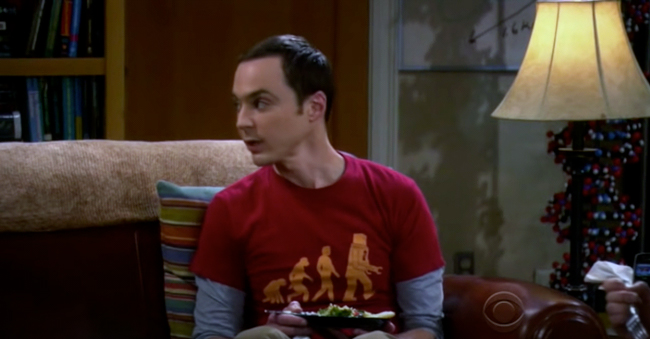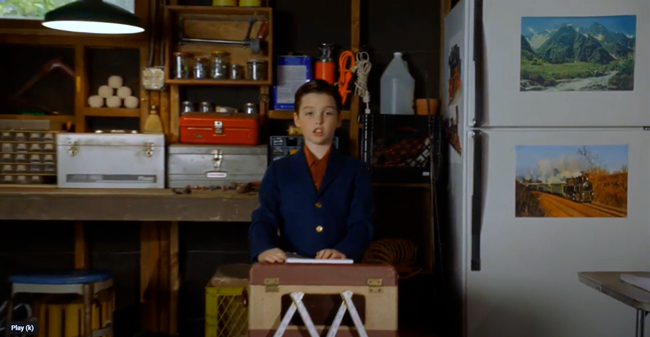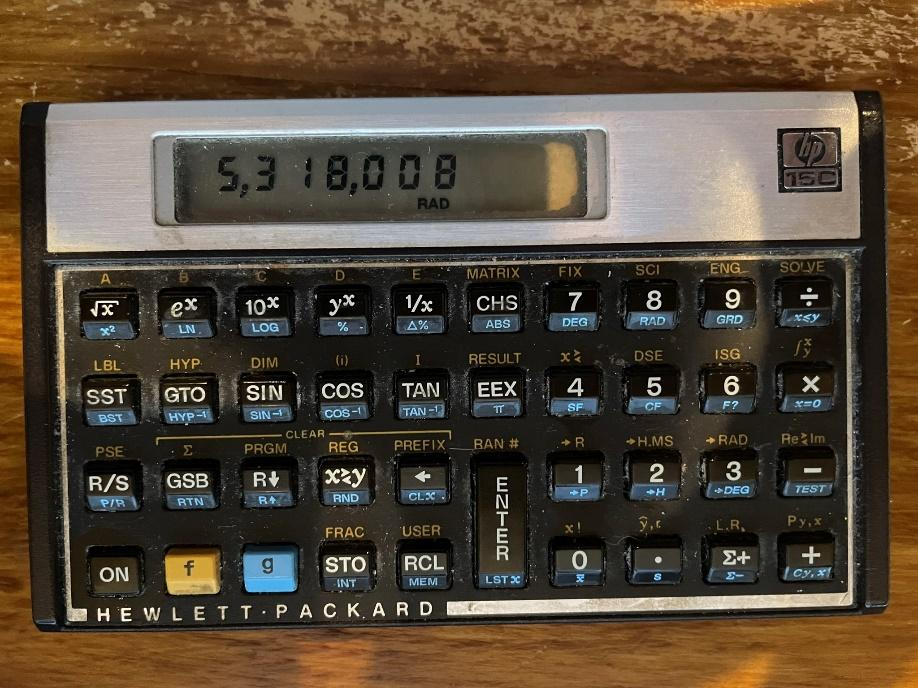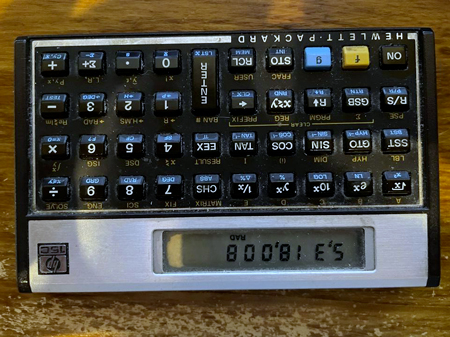## Wizard Recommends

Last Updated: April 28, 2022

# Reasons why 73 is the best number

I don’t claim that 73 is the best number. In fact, I don’t even claim it to be the best integer. However, Sheldon of the Big Bang Theory argued why it was the best number. Not only does that make for a great trivia question but deserves a careful analysis.

Before I go further, here is a link to a clip where this is discussed on the Big Bang Theory.Here are Sheldon’s reasons why 73 is the best number, or the “Chuck Norris of numbers” as Leonard put it.

1. 73 is 21st prime number. It’s mirror, 37, is the 12th prime number (the mirror of 21).
2. 7*3 = 21. As previously mentioned, 73 is the 21st prime number.
3. 73 = 64 + 8 + 1 = 2^6 + 2^3 + 2^1. Represented in binary, 73 = 1001001, which is a palindrome (expressed the same way forwards as backwards).

Here are some other reasons why 73 might be considered the best number, which Sheldon didn’t mention, but Wikipedia does.

1. Not only is 73 a star number , but so is it’s mirror 37. In fact, there are no star numbers between 37 and 73.
2. Not only is 73 a palindrome in base 2, but also base 8. 73 = 8^2 + 8^1 + 8^0 = 111.
3. 7^3 = 343, another prime.In the star figure above (from Wikipedia) , there are 37 green circles and 73 blue ones.

Wikipedia mentions some other properties of the number 73, but they are so esoteric, I won’t get into them. However, many do involve prime numbers. This makes for a perfect connection to Sheldon’s first sermon on prime numbers in his church of mathology on the prequel Young Sheldon.I can’t help but comment on Raj’s argument that 5,318,008 is the best number. Here is what it looks like right-side up.However, here it is upside-down.In closing, this is a rare time I disagree with Sheldon. There can be little debate the two best numbers are e and π, preferably in that order. However, if we must limit ourselves to integers, I’m going to go with 153. I will explain why next week, unless I think of something better to write about.

Until then, may be the odds be in your favor.